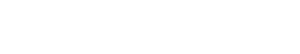# Correlation

Correlation is used to refer to the relationship between two or more variables. This relationship can be positive, negative, or none at all. In other industries, correlation may have a different meaning. For example, in statistics, correlation is a measure of how two variables are related to each other. Correlation can be used to understand the strength of the relationship between two variables, as well as the direction of the relationship.

## Pearson Correlation Coefficient

There are many ways to calculate correlation, but the most common method is to use the Pearson Correlation Coefficient. This coefficient measures the linear relationship between two variables. The coefficient is calculated by taking the covariance of the two variables and dividing it by the product of their standard deviations.

The Pearson Correlation Coefficient can range from -1 to 1. A value of -1 indicates a perfect negative linear relationship between the two variables, meaning that as one variable increases, the other decreases. A value of 1 indicates a perfect positive linear relationship between the two variables, meaning that as one variable increases, the other also increases. A value of 0 indicates that there is no linear relationship between the two variables.

It is important to note that correlation does not necessarily imply causation. Just because two variables are correlated does not mean that one variable is causing the other to change. Correlation can be affected by many factors, so it is important to consider all of the data before making any conclusions.

When looking at data, it is often helpful to compare correlation to other similar terms, such as association and dependence. Association is used to describe the relationship between two or more variables, but it does not necessarily imply a cause-and-effect relationship. Dependence occurs when one variable is determined by another variable; in other words, the dependent variable cannot exist without the independent variable.Unlock the power of actionable insights with AI-based natural language processing.